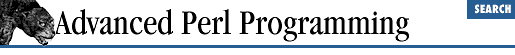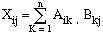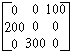#Chapter 2Implementing Complex Data Structures## 2.2 Example: Matrices

Before we embark on this example, you must know that if you really want a good efficient implementation of matrices, you should check out the PDL module (Perl Data Language) from CPAN.

To gain a better understanding of different matrix representations, we will write routines to construct these structures from a data file and to multiply two matrices. The file is formatted as follows:

```MAT1
1  2  4
10 30 0

MAT2
5  6
1  10```

Each matrix has a label and some data. We use these labels to create global variables with the corresponding names (@MAT1 and @MAT2).

An array of arrays is the most intuitive representation for a matrix in Perl, since there is no direct support for two-dimensional arrays:

```@matrix = (
[1, 2, 3],
[4, 5, 6],
[7, 8, 9]
);
# Change 6, the element at row  1, column 2 to 100
\$matrix = 100;```

Note that @matrix is a simple array whose elements happen to be references to anonymous arrays. Further, recall that \$matrix is a simpler way of saying \$matrix->.

Example 2.1 reads the file and creates the array of arrays structure for each matrix. Pay particular attention to the push statement (highlighted); it uses the symbolic reference facility to create variables (@{\$matrix_name}) and appends a reference to a new row in every iteration. We are assured of newly allocated rows in every iteration because @row is local to that block, and when the if statement is done, its contents live on because we squirrel away a reference to the array's value. (Recall that it is the value that is reference counted, not the name.)

#### Example 2.1: Reading a Matrix from a File

```sub matrix_read_file {
my (\$filename) = @_;
open (F, \$filename) || die "Could not open \$filename: \$!";
while (\$line = <F>) {
chomp(\$line);
next if \$line =~ /^\s*\$/; # skip blank lines
if (\$line =~ /^([A-Za-z]\w*)/) {
\$matrix_name = \$1;
} else {
my (@row) = split (/\s+/, \$line);
push (@{\$matrix_name}, \@row;) # insert the row-array into
# the outer matrix array
}
}
close(F);
}```

Now let us use this array-of-arrays structure to multiply two matrices. In case you have forgotten how matrix multiplication works, the product of two matrices Amn (m rows, n columns) and Bnp is defined asThe element (i, j) of the matrix product is the sum of successive pairs of elements taken from the ith row of A and the jth column of B. Translated into Perl, it looks like Example 2.2.

#### Example 2.2: Matrix Multiply

```sub matrix_multiply {
my (\$r_mat1, \$r_mat2) = @_;       # Taking matrices by reference
my (\$r_product);                  # Returing product by reference
my (\$r1, \$c1) = matrix_count_rows_cols (\$r_mat1);
my (\$r2, \$c2) = matrix_count_rows_cols (\$r_mat2);
die "Matrix 1 has \$c1 columns and matrix 2 has \$r2 rows."
. " Cannot multiply\n" unless (\$c1 == \$r2);
for (\$i = 0; \$i < \$r1; \$i++) {
for (\$j = 0; \$j < \$c2; \$j++) {
\$sum = 0;
for (\$k = 0; \$k < \$c1; \$k++) {
\$sum += \$r_mat1->[\$i][\$k] * \$r_mat2->[\$k][\$j];
}
\$r_product->[\$i][\$j] = \$sum;
}
}
\$r_product;
}

sub matrix_count_rows_cols {  # return number of rows and columns
my (\$r_mat)  = @_;
my \$num_rows = @\$r_mat;
my \$num_cols = @{\$r_mat->}; # Assume all rows have an equal no.
# of columns.
(\$num_rows, \$num_cols);
}```

`matrix_multiply` takes two matrices by reference. A single element is obtained as \$r_mat->[\$i][\$j], and a single row is obtained as \$r_mat->.

### 2.2.1 Hash of Hashes Representation

If the matrix is large and sparse (only a few elements have nonzero values), a hash of hashes is likely a more space-efficient representation. For example, the matrixcan be built like this:

```\$matrix{0}{2} = 100;
\$matrix{1}{0} = 200;
\$matrix{2}{1} = 300;```

The code above creates a hash table, %matrix, which maps a row number to a nested hash. Only rows with nonzero elements are represented. Each nested hash maps a column number to the actual value of the element at that row and column. Again, only columns with nonzero values in that row are represented. Of course, we have to store the total number of rows and columns separately, since unlike the array representation, these numbers are not implicit. Since %matrix is a hash, they can be stored as \$matrix{rows} and \$matrix{cols}. Because hash indices are strings, this approach is efficient only if the matrix is large and sparse.

To make the matrix routines developed in the previous section work for this new representation, it might seem a simple matter to convert all square brackets to braces. True, it'll work, but there's a subtle problem. Let's say that the entire third row of a matrix is zero-valued (so there's no entry for \$r_mat->{2}). Now, if you do this:

`\$element = \$r_mat->{2}{3}; `

Perl automatically creates an entry for \$r_mat->{2}, and hangs a hash reference off this entry. (The nested hash table is not created.) Thus the very act of examining an element gobbles up space, which is what we had hoped to avoid in the first place when we chose the hash of hashes representation. To prevent this, we have to check for the presence of a hash element with exists before retrieving it, as shown next:

```if ((exists \$r_mat->{\$row}) && (exists \$r_mat->{\$row}{\$col})) {
....```

### 2.2.2 Other Matrix Representations

If the columns are sparse but the rows are well represented, you could choose an array of hashes structure. It is possible to store a matrix even more economically in terms of space, using a single hash table, at the expense of more complex code. If you imagine the matrix as a grid and number each cell of the grid consecutively, any cell can then be identified with exactly one unique number. So in a matrix with 10 rows and 5 columns, the element (8, 4) will have the number 38 (7 * 5 + 3) and hence can be referred to as \$r_mat->{38}. We actually use this scheme in Chapter 15, GUI Example: Tetris (though that usage is more for convenience than for saving space). The choice of data structure depends on the size of the matrices, performance, and coding convenience.

Changing the data structure of a program clearly ends up changing all code that depends on it. To contain the amount of changes required (should the structure change), it is always a good idea to have only a small set of procedures that know the structure. For example, if you had procedures such as `create_matrix( )`, `get_element(mat,i,j)`, and `set_element(mat,i,j)`, other procedures do not have to know the internal representation. Coding for change is often better than coding for run-time efficiency. We'll discuss this approach a great deal more in Chapter 7.2.1 User-Defined Structures2.3 Professors, Students, Courses